Courses

# Deducing the Machine Performance Electrical Engineering (EE) Notes | EduRev

## Electrical Engineering (EE) : Deducing the Machine Performance Electrical Engineering (EE) Notes | EduRev

The document Deducing the Machine Performance Electrical Engineering (EE) Notes | EduRev is a part of the Electrical Engineering (EE) Course Electrical Machines.
All you need of Electrical Engineering (EE) at this link: Electrical Engineering (EE)

Deducing the machine performance.

From the equivalent circuit, many aspects of the steady state behavior of the machine can be deduced. We will begin by looking at the speed-torque characteristic of the machine.

We will consider the approximate equivalent circuit of the machine. We have reasoned earlier that the power consumed by the ’rotor-portion’ of the equivalent circuit is the power transferred across the air-gap. Out of that quantity the amount dissipated in Rr is the rotor copper loss and the quantity consumed by Rr (1 − s)/s is the mechanical power developed. Neglecting mechanical losses, this is the power available at the shaft. The torque available can be obtained by dividing this number by the shaft speed.

The complete torque-speed characteristic

In order to estimate the speed torque characteristic let us suppose that a sinusoidal voltage is impressed on the machine. Recalling that the equivalent circuit is the per-phase representation of the machine, the current drawn by the circuit is given by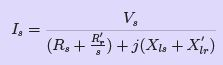(14)
where Vs is the phase voltage phasor and Is is the current phasor. The magnetizing current is neglected. Since this current is ﬂowing through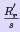, the air-gap power is given by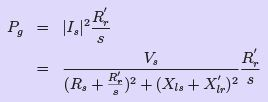(15)
The mechanical power output was shown to be (1 − s)P(power dissipated in Rr /s). The torque is obtained by dividing this by the shaft speed ωm.Thus we have,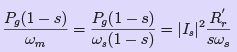(16)
where ωs is the synchronous speed in radians per second and s is the slip. Further, this is the torque produced per phase. Hence the overall torque is given by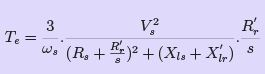(17)
The torque may be plotted as a function of ‘s’ and is called the torque-slip (or torquespeed, since slip indicates speed) characteristic — a very important characteristic of the induction machine. Eqn. 17 is valid for a two-pole (one pole pair) machine. In general, this expression should be multiplied by p, the number of pole-pairs. A typical torque-speed characteristic is shown in ﬁg. 22. This plot corresponds to a 3 kW, 4 pole, 60 Hz machine.
The rated operating speed is 1780 rpm.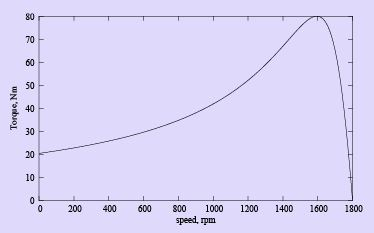Figure 22: Induction machine speed-torque characteristic

We must note that the approximate equivalent circuit was used in deriving this relation.
Readers with access to MATLAB or suitable equivalents (octave, scilab available free under GNU at the time of this writing) may ﬁnd out the diﬀerence caused by using the ‘exact’ equivalent circuit by using the script found here. A comparison between the two is found in the plot of ﬁg. 23. The plots correspond to a 3 kW, 4 pole, 50 Hz machine, with a rated speed of 1440 rpm. It can be seen that the approximate equivalent circuit is a good approximation in the operating speed range of the machine. Comparing ﬁg. 22 with ﬁg. 23, we can see that the slope and shape of the characteristics are dependent intimately on the machine parameters.

Further, this curve is obtained by varying slip with the applied voltage being held constant. Coupled with the fact that this is an equivalent circuit valid under steady state, it implies that if this characteristic is to be measured experimentally, we need to look at the torque for a given speed after all transients have died down. One cannot, for example, try to obtain this curve by directly starting the motor with full voltage applied to the terminals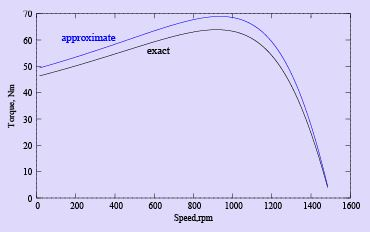Figure 23: Comparison of exact and approximate circuit predictions and measuring the torque and speed dynamically as it runs up to steady speed.

Another point to note is that the equivalent circuit and the values of torque predicted is valid when the applied voltage waveform is sinusoidal. With non-sinusoidal voltage waveforms, the procedure is not as straightforward.

With respect to the direction of rotation of the air-gap ﬂux, the rotor maybe driven to higher speeds by a prime mover or may also be rotated in the reverse direction. The torque-speed relation for the machine under the entire speed range is called the complete speed-torque characteristic. A typical curve is shown in ﬁg. 7.1 for a four-pole machine, the synchronous speed being 1500 rpm. Note that negative speeds correspond to slip values greater than 1, and speeds greater than 1500 rpm correspond to negative slip. The plot also shows the operating modes of the induction machine in various regions. The slip axis is also shown for convenience.

Restricting ourselves to positive values of slip, we see that the curve has a peak point.

This is the maximum torque that the machine can produce, and is called as stalling torque.

If the load torque is more than this value, the machine stops rotating or stalls. It occurs at a slip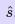, which for the machine of ﬁg. 7.1 is 0.38. At values of slip lower than, the curve falls steeply down to zero at s = 0. The torque at synchronous speed is therefore zero. At values of slip higher than s =, the curve falls slowly to a minimum value at s = 1. The torque at s = 1 (speed = 0) is called the starting torque.

The value of the stalling torque may be obtained by diﬀerentiating the expression for torque with respect to zero and setting it to zero to ﬁnd the value of. Using this method,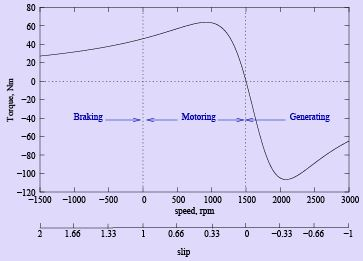Figure 24: Complete speed-torque characteristic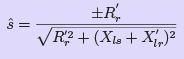(18)
Substitutinginto the expression for torque gives us the value of the stalling torque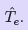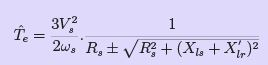(19)
the negative sign being valid for negative slip.

The expression shows thatis the independent of Rr, whileis directly proportional to Rr . This fact can be made use of conveniently to alter. If it is possible to change Rr , then we can get a whole series of torque-speed characteristics, the maximum torque remaining constant all the while. But this is a sub ject to be discussed later.
We may note that if Rr′ is chosen equal to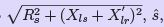becomes unity, which means that the maximum torque occurs at starting. Thus changing of Rr , wherever possible can serve as a means to control the starting torque.
While considering the negative slip range, (generator mode) we note that the maximum torque is higher than in the positive slip region (motoring mode).

Operating Point

Consider a speed torque characteristic shown in ﬁg. 25 for an induction machine, having the load characteristic also superimposed on it. The load is a constant torque load i.e.,the torque required for operation is ﬁxed irrespective of speed.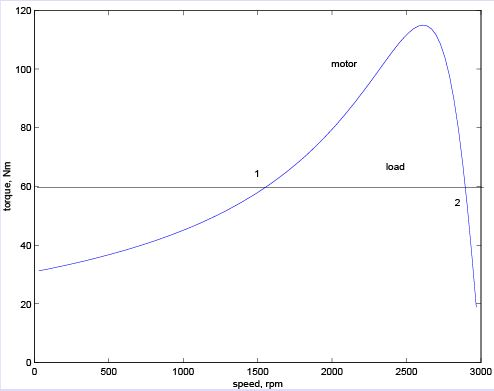Figure 25: Machine and load characteristics

The system consisting of the motor and load will operate at a point where the two characteristics meet. From the above plot, we note that there are two such points. We therefore need to ﬁnd out which of these is the actual operating point.

To answer this we must note that, in practice, the characteristics are never ﬁxed; they change slightly with time. It would be appropriate to consider a small band around the curve drawn where the actual points of the characteristic will lie. This being the case let us considers that the system is operating at point 1, and the load torque demand increases slightly. This is shown in ﬁg. 26, where the change is exaggerated for clarity. This would shift the point of operation to a point 1 at which the slip would be less and the developed torque higher.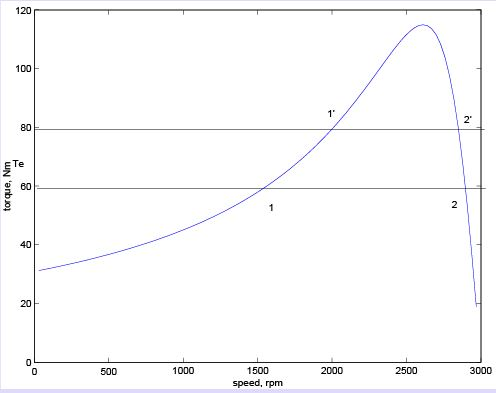Figure 26: Stability of operating point

The diﬀerence in torque developed △Te, being positive will accelerate the machine. Any overshoot in speed as it approaches the point 1 will cause it to further accelerate since the developed torque is increasing. Similar arguments may be used to show that if for some reason the developed torque becomes smaller the speed would drop and the eﬀect is cumulative. Therefore we may conclude that 1 is not a stable operating point.

Let us consider the point 2. If this point shifts to 2, the slip is now higher (speed is lower) and the positive diﬀerence in torque will accelerate the machine. This behavior will tend to bring the operating point towards 2 once again. In other words, disturbances at point 2 will not cause a runaway eﬀect. Similar arguments may be given for the case where the load characteristic shifts down. Therefore we conclude that point 2 is a stable operating point.

From the foregoing discussions, we can say that the entire region of the speed-torque characteristic from s = 0 to s =is an unstable region, while the region from s =to s = 0 is a stable region. Therefore the machine will always operate between s = 0 and s =.

Modes of Operation

The reader is referred to ﬁg. 7.1 which shows the complete speed-torque characteristic of the induction machine along with the various regions of operation.
Let us consider a situation where the machine has just been excited with three phase supply and the rotor has not yet started moving. A little reﬂection on the deﬁnition of the slip indicates that we are at the point s = 1. When the rotating magnetic ﬁeld is set up due to stator currents, it is the induced emf that causes current in the rotor, and the interaction between the two causes torque. It has already been pointed out that it is the presence of the non-zero slip that causes a torque to be developed. Thus the region of the curve between s = 0 and s = 1 is the region where the machine produces torque to rotate a passive load and hence is called the motoring region. Note further that the direction of rotation of the rotor is the same as that of the air gap ﬂux.

Suppose when the rotor is rotating, we change the phase sequence of excitation to the machine. This would cause the rotating stator ﬁeld to reverse its direction — the rotating stator mmf and the rotor are now moving in opposite directions. If we adopt the convention that positive direction is the direction of the air gap ﬂux, the rotor speed would then be a negative quantity. The slip would be a number greater than unity. Further, the rotor as we know should be ”dragged along” by the stator ﬁeld. Since the rotor is rotating in the opposite direction to that of the ﬁeld, it would now tend to slow down, and reach zero speed.

Therefore this region (s > 1) is called the braking region. (What would happen if the supply is not cut-oﬀ when the speed reaches zero?) There is yet another situation.

Consider a situation where the induction machine is operating from mains and is driving an active load (a load capable of producing rotation by itself ). A typical example is that of a windmill, where the fan like blades of the wind mill are connected to the shaft of the induction machine. Rotation of the blades may be caused by the motoring action of the machine, or by wind blowing. Further suppose that both acting independently cause rotation in the same direction. Now when both grid and wind act, a strong wind may cause the rotor to rotate faster than the mmf produced by the stator excitation. A little reﬂection shows that slip is then negative. Further, the wind is rotating the rotor to a speed higher than what the electrical supply alone would cause. In order to do this it has to contend with an opposing torque generated by the machine preventing the speed build up.

The torque generated is therefore negative. It is this action of the wind against the torque of the machine that enables wind-energy generation. The region of slip s > 1 is the generating mode of operation. Indeed this is at present the most commonly used approach in wind-energy generation. It may be noted from the torque expression of eqn. 17 that torque is negative for negative values of slip.

Offer running on EduRev: Apply code STAYHOME200 to get INR 200 off on our premium plan EduRev Infinity!

61 docs|20 tests

,

,

,

,

,

,

,

,

,

,

,

,

,

,

,

,

,

,

,

,

,

;